# How to make inductance meter using Arduino

This circuit gives accurate reading to all types of an inductor, we can measure unknown inductor value using this. Arduino inductor meter.

BeginnerFull instructions provided1 hour9,477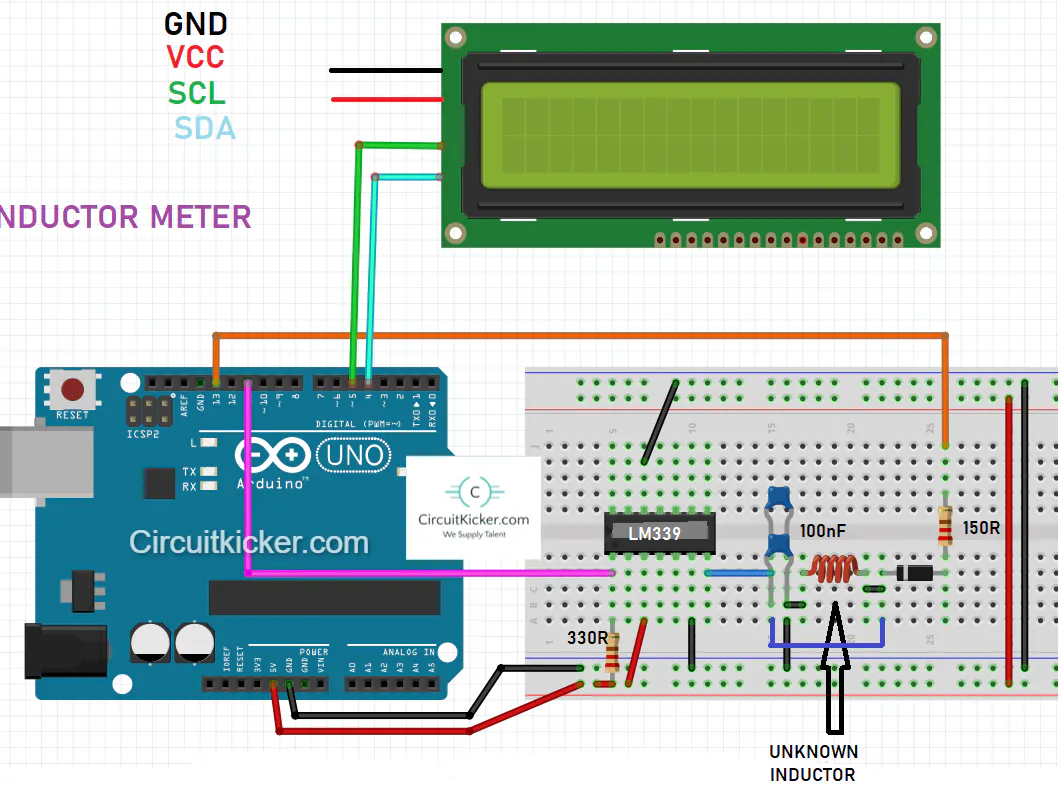## Things used in this project

### Hardware components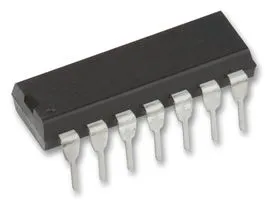×1Arduino UNO
×1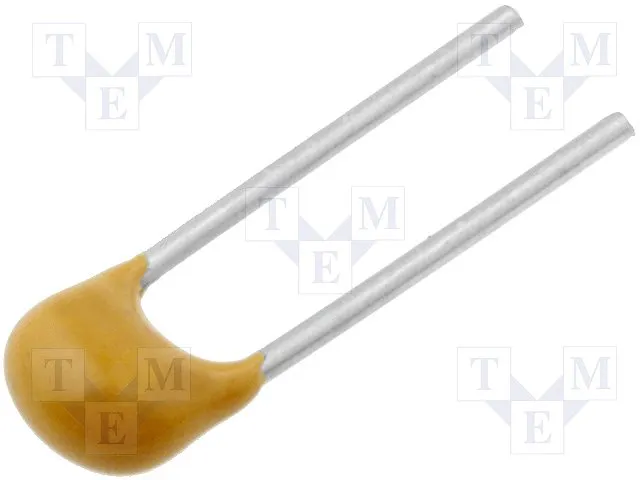Capacitor 100 nF
×1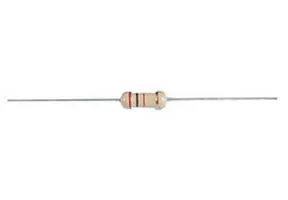Through Hole Resistor, 390 ohm
×1Resistor 1k ohm
×1LED (generic)
×1

### Software apps and online servicesJLCPCB EasyEDAArduino IDE

### Hand tools and fabrication machines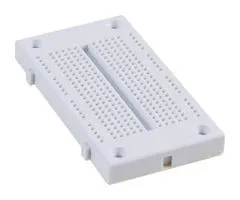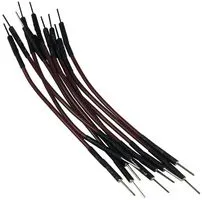10 Pc. Jumper Wire Kit, 5 cm Long

## Code

### inductance meter Arduino code

C/C++
```//Circuit and code is uploaded by Circuitkicker.com
//some important things:
// 1) If using Serial monitor to read the value of inductor then select 115200 boud.
// 2) Adjust the value by using fixed inductor by changing 2.E-7 line.
// 3) We will get acuurate readings because of many iteratations.

#include <Wire.h>
#include <LiquidCrystal_I2C.h>
LiquidCrystal_I2C lcd(0x27,20,4);  //sometimes the adress is not 0x3f. Change to 0x27 if it doesn't work.

//13 is the input to the circuit (connects to 150ohm resistor), 11 is the comparator/op-amp output.
double pulse, frequency, capacitance, inductance, inductance_mH;
void setup(){
lcd.init();
lcd.backlight();
Serial.begin(115200);
pinMode(11, INPUT); //Input from the comparator output
pinMode(13, OUTPUT);//output through a 150 ohm resistor to thr LC circuit
Serial.println("HELLO SIR");
delay(1000);
Serial.println("Circuitkicker.com");
delay(2500);
}
void loop(){
digitalWrite(13, HIGH);
delay(5);//give some time to charge inductor.
digitalWrite(13,LOW);
delayMicroseconds(100); //make sure resination is measured
pulse = pulseIn(11,HIGH,5000);//returns 0 if timeout
if(pulse > 0.1){ //if a timeout did not occur and it took a reading:

capacitance = 2.E-7; // <- insert value here

frequency = 1.E6/(2*pulse);
inductance = 1./(capacitance*frequency*frequency*4.*3.14159*3.14159);
inductance *= 1E6; //note that this is the same as saying inductance = inductance*1E6
inductance_mH = inductance/1000;
//Serial print
Serial.print("High for uS:");
Serial.print( pulse );
Serial.print("\tfrequency Hz:");
Serial.print( frequency );
Serial.print("\tinductance uH:");
Serial.println( inductance );
delay(10);

//LCD print
lcd.clear();
lcd.setCursor(0,0);
lcd.print("uH Inductance mH");
lcd.setCursor(0,1);
lcd.print(inductance);
lcd.setCursor(10,1);
lcd.print(inductance_mH);
delay(10);
}
}
```

## Credits

### sagar saini

35 projects • 21 followers
I am Sagar Saini. A PCB engineer and project manager in Farm Network Group. Works for National agencies.